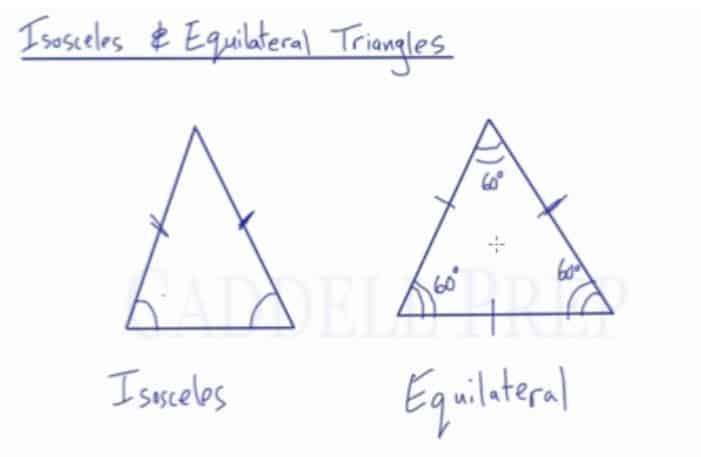In this video, we are going to look at isosceles and equilateral triangles.

Isosceles Triangles: Isosceles triangles have at least two congruent sides. Two sides of an isosceles triangle are the same length, so the angles directly across from them are the same measure.

Equilateral Triangles: Equilateral triangles have all three sides congruent. Since all the sides are the same length, all the angles are the same measure. A triangle has a total of$180\textdegree$, so each angle must equals$60\textdegree$.Video-Lesson Transcript

Let’s take a look at isosceles and equilateral triangles.

These are two important triangles.

Isosceles triangles have two sides that are the same. If the two sides are the same, then the angles across from them are also the same.

Here is an equilateral triangle. Equilateral means all equal. So, all the sides are the same length and all the angles are the same as well. And in a triangle, angles have to add up to$180^{\circ}$. This means that each angle is$60^{\circ}$.

For isosceles, two angles that are the same and we know that the sides across from them are also equal. And vice versa.

While in an equilateral triangle, if we have two angles that are$60^{\circ}$, then we know that the third angle is also$60^{\circ}$. And we also know that all the sides are the same.